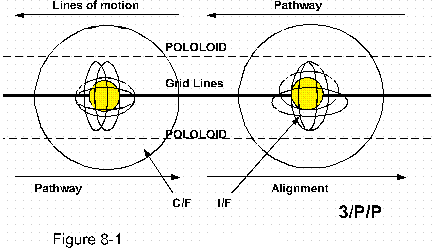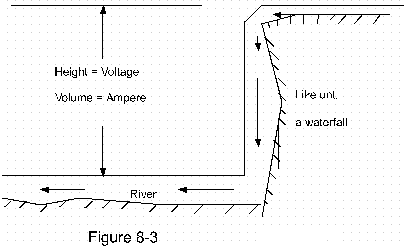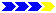# NATURE AND ORDER OF FORCE

CHAPTER 8INDEX TO OTHER PAGES

WHAT  IS  ELECTRICITY?

1. That invisible power, the bite of which splits a tree into halves, arks through the night sky with a splendor like none other, burns to a crisp, illuminates our homes, and provides man with power to ease his burdens.  What is this electricity that can do all these things the nature of which has remained a secret for so many centuries?

2. Nations have come and gone, one wise man after the other, and even knowledge increased, and tools were given us wherewith to better research a thing, and yet the secret remained a secret.  Not knowing the nature of things, yet determined to succeed man replaced old errors with new errors and the secret remained.

3. This age-old secret has now however been granted us, and I will manifest the same with both its nature as well as to its form.  This was granted me from our Creator for the benefit of all mankind, and to that end have I labored to define this marvelous secret in terms everyone should be able to understand.

4. The nature of what electricity is, and also of what magnetism is, can be put into one word namely; "motion".  But this word on its own does not give us an understanding of its phenomena.  We therefore should add to that word, that electricity as well as magnetism are specific formations of motion.

5. And with that word "motion," we refer to specific coordinates of movements, conducted and set forth by the countless engines of nature, namely; atoms.

6. In the nature of our nature we find a number of forces to which we have given names, such as; magnetic force, electrical force, gravitational force, thermal force, the force of the wind, the force of impact, and more such.  But which of these if any is real or fundamental, to say from this one force all others may be born?

7. This one force then is "motion".  But with that we are right back at asking what specific formation of motion is most fundamental in terms of force, to which the answer is: Magnetism.  It is magnetic force, the magnetic force of all celestial bodies and systems of bodies, which is first, and fundamental to any force that may be named.

 MAGNETISM Magnetic force                                Electrical force E/M Waves              Gravitational force                 Centrifugal force The wind             Rain,            Clouds,            Temperature,           Tides Structures                  States of matter                   Celestial systems
1. To define an order of things, magnetism is the most fundamental coordinate of force, its typical pattern is the single circle of motion turned over by one half turn to present the figure of eight.

2. This particular pattern then may be expressed as "stationary".  The term "Magnetism" then becomes a general expression, while "magnetic" as such is in reference to the force thereof, or to any other specific of the same.

3. Electrical force here is a derivative of magnetism, as indeed electricity is none other than magnetism, and electrical force none other than a "magnetic force".  The two forces of magnetism consequently are (as listed) Magnetic, and electric.

4. The next derivatives of magnetism, in an order further removed, such as gravity, centrifugal impact, and the E-M wave spectrum.  After this follow such forces as the wind, or the tides, in the powers set forth by the movement of the earth the sun and moon.

5. None of which would subsist if it were not for the foremost magnetic force by which they may be obtained and maintained.

6. Accordingly, even though the wind is not directly from magnetic force, it is indirectly so, or fundamentally so.  And the same goes for the states of matter which by structuring come to their appearance, be it a simple pebble or a massive celestial system.

7. In previous chapters we outlined the "magnitudes" of nature, which are also called the "orders" of nature.  These were named after the physical nomenclature of nature, "matter, motion, and coordinate," which in turn divided the physical nature in two material groups, as we called them the "first" and "second" magnitudes (or orders), with the coordinate as the third thereof.

8. These very same orders can then also be called "the orders of magnetism", since magnetism as such is "the" fundamental coordinate of movement, and "movement", is "motion", while motion is again the one power of the physical realm.

9. In this reference then, to say, "the orders of magnetism", we place the emphasis on forces of which there are six in the three orders.  Each order has motion as its force, which always comes in two directions, linear and angular.

10. While in the greater then" (third) order these are; magnetic force as the linear, and electrical force as the angular, while in the first two orders they are named after their direction.

Orders of magnetism

1. Another question might be - how do we create an order or a principle by which to behold or define something?  The idea is to find a means of reference by way of distinctions.  And to do this with magnetism, we begin with the atom.

2. How therefore may we plot the atom into points of distinction that indeed serve to show its principle of operation, and which at the same time is in harmony with the grand scheme of things?The atoms in the illustrations are shown as planetary systems, mainly to humor mankind, the reality is quite different.

1. Reference figure 8-1;  This is done in this way: We begin by striking a line over the core, which establishes, "a stationary center", that may also be called a grid line."  Next we strike a line along the perimeter of the atom at two sides, and place arrows upon them to denote movement and the direction of movement.

2. These lines therefore we call "lines of motion", or power-lines, each of which come to serve and establish the "pathways" of nature.  The three lines so noted thus establish matter motion and coordination, or base, movement, and formation.

3. The grid lines are very real in nature bonding atoms into molecules and molecules into greater structures.  The power-lines also are very real in nature since these, as the pathways of nature, serve to transpose waves, and the lines of motion we call "lines of force".

4. Here thus we find again that fundamental principle where one is in three and three in one.  Neither one of the power lines could exist on its own, nor even present power or force, since each one serves also as the "reactance" of the other.

5. And to give an example: If the atom wished to present a push of power towards the left from its top (Figure 8-1) it would fail to present any, since it is not properly based.  Something must hold the atom in place so that its power-line may indeed be valid.

6. As it is now, it would push the atom back; the reaction to the action is not there.  But with two power-lines one precisely over against the other in equal proportions, one is reacted upon by the other.

7. But still neither of these would be valid if it were not for their common base (nucleus, gridline (base line) upon which they may structure themselves.  Take away the base, and the power is gone as well.  Or, remove the power, and even the base is no longer valid as such.

8. We are in other words are right back at the principle of three, without any one leg neither of three would be, and to have one is to have all three, or have nothing.

Pololoid

1. In addition to the foregoing, a means of establishing a scale for motion may be set which I called, "Pololoid".  This also is by a three-point reference mode set in between the two power-lines as the single movement of power, and the nucleus as the base.

2. The purpose of Pololoid is to account the balance, and/or resistance of motion to any and all factors known in nature.

3. Pololoid may for example determine the expansion and contraction of the atom in size, as well as temperature, velocity and structural integrity of all states of matter.  Pololoid is actually much more than just these things, and I have set aside a chapter devoted to just it.

Alignments, and fields of force

1. Alignment is simply the interaction of the motion of one atom to that of the next to establish what I call the "pathways" of nature, lines of motion along which electrical and magnetic formations have their power, and the tracks along which waves are found to travel.

2. Nature starts out with intrinsic motion, the spinning motion. (Not really the first, but fundamental on the atoms)   (Figure 8-2).  The first of the forces, or fields of force, is that force extended from the core that extends beyond the perimeters, and we shall call it; The inner field, (I/F).

3. Next, that movement as a field of force may be called: Circular field.  (C/F)  These are the two fields, or forces of the first magnitude of magnetism.

4. When therefore we come to combine inner fields one to the next, we end up with grids that are literally endless in the realm of nature, which makes for all the structures in nature.  And to give them a name, we could say "lines of", or simply as we said earlier, "the grid lines, or base lines" of nature.

5. It is along these lines of motion that sound is found to travel, and as whole grids these lines serve most excellent to backup (base) all the fundamental powers of force in nature.

6. Circular fields then in multiples become: "Unit magnetic lines", or field lines of force, which more appropriate are called pathways, or as I often call them, nature's "general" lines of motion.  These two are the two forces or fields of force belonging to the second magnitude of nature, since they are the multiples of the first.

Third order forces

1. When the fundamental forces of the two orders of nature are combined to greater lengths and magnitudes, we come to forces that are "greater then" forces, which are, "magnetic", and "electric".

2. Still another term that I use in the stead of "third order", is to say; the "overall" order of nature, like; "overall magnetic field", or "overall magnetic", meaning, as belonging to the third order.

3. Essentially, magnetism has the aurora of being attractive, and in that sense all of nature is magnetism, for when any two atoms interact to join in unity to a greater formation, so is magnetism.  And magnetism as motion interactive comes to the same end.

The flow of electricity

1. Electricity can be had from any substance or place in nature.  This is done merely by creating a certain opposition within that motion of nature (general motion) that is everywhere within and upon all media (substance).  And so the general motion of nature may be likened to a river flowing down to sea.

2. When therefore we install a dam in that river, we establish; a 'potential difference' (Figure 8-3), which is in fact a "potential" of the flow, and can be utilized to any means for power that we may require.

3. The potential will of course be proportional to the height of the dam, as the height (voltage) from which the water is to fall, and/or the volume (ampere) of the water going down the river.1. In all reality, electricity is simply the potential opposition we drive in the general motion of nature utilizing "turns of motion" (angular movement).  Or as I said; a magnetic field of force twisted into a continues caption of figure eight's, in which case it not only becomes, but is in fact; an angular, or rotational magnetic force.

2. And the movement in two opposite directions as the power to extend into the pathways of natureNext page